# Chapter 8 : molecular physical chemistry – operators

Operators can be applied to the wave functions and respect the equation of Schrödinger. The operator inversion Î is an operator such as, if a central symmetry can be found,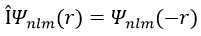For instance, the orbital s have a centre of symmetry. We say that this state is even. If we apply the inversion operator to this orbital s, the sign of the orbital is still unchanged at the opposite side of the atom (i.e. obtained by central symmetry).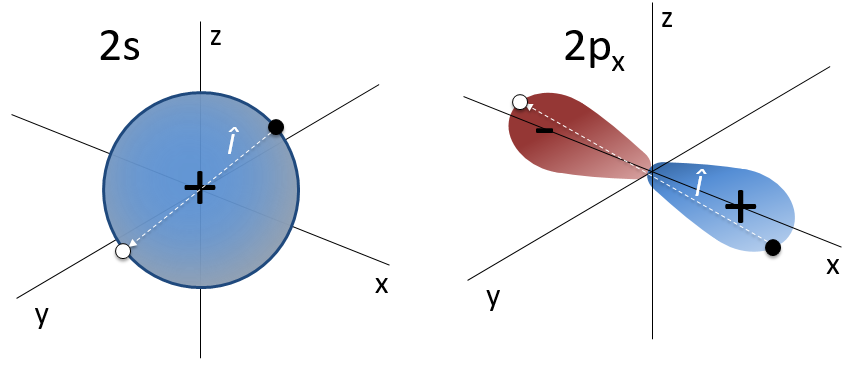The orbital p is odd because if we look at one point in the positive part of the orbital and apply the symmetry, we are now in the negative lobe of the orbital. The d orbitals are even. If fact, we have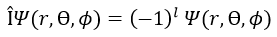The operator Î can also be applied to electrons to obtain the opposite spin (up à down).

Angular moments

The angular moment L is the cross product of two vectors r and p. The cross product or vector product is a binary operation on two vectors and is denoted by the symbol ×. Given two linearly independent vectors a and b, the cross product, a × b, is a vector c that is perpendicular to both and therefore normal to the plane containing them (two linear vectors always share a plane).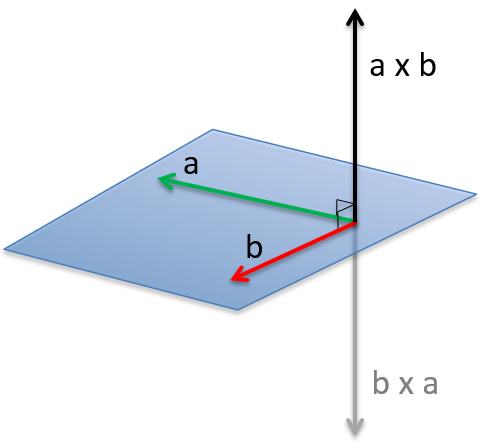The intensity of the new vector is a × b = ∣a∣∣b∣ sin n (with n the unity vector pointing in the good direction) and the direction of the cross product is given by the rule of the right hand: the index stands points in the direction of the first vector and the middle finger points in the direction of the second vector. If you put your thumb perpendicular to the two other fingers (as to show your approval), it points the direction of the cross product. It can be necessary to rotate your hand strangely to get the good directions, for instance to obtain the b x a product from above.

In quantum mechanics, p is an operator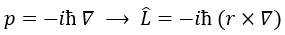We have thus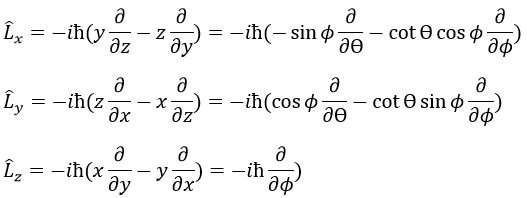Note that Lz does not depend on and there is thus a symmetry around the z axis for this operator. If we apply the operator Lz on the angular wave function, we obtain the wave function multiplied by ћm: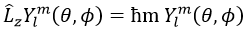It means that the angular wave function is a proper function of the operator Lz. It is also the case with the operator L2.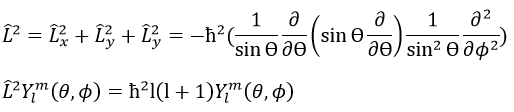We can thus simultaneously determine the proper values of L2 (2l(l+1)), characteristic of the angular moment L, and of Lz (=ћm), the projection of L on the z axis, for fixed values of the quantic numbers.

The proper value of an operator is the integral of the wave function multiplied by the operator acting on the wave function: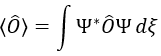The length of the vector L and of its projections on the axes.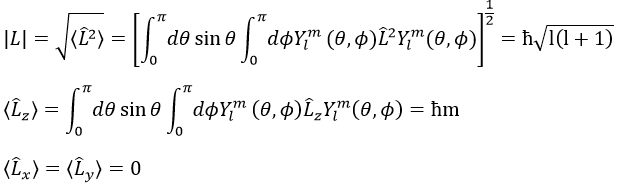For instance, for l=1, we can draw L and its projection as a function of m. The length of L doesn’t depend on m but the projection on the axis z does.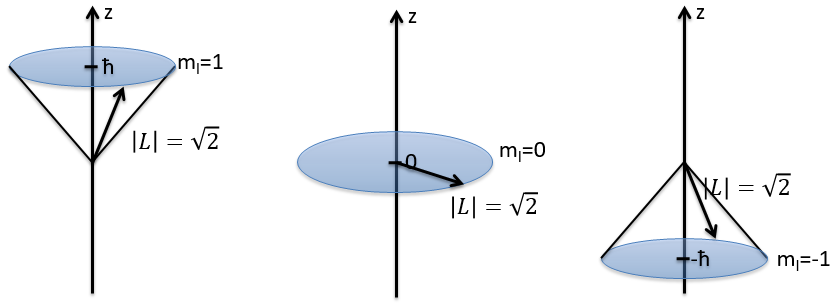L2 and Lz commute: two operators that commute have the same wave function. The commutativity means that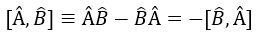It is indeed the case: [L2,Lz]=0 and it is not the case between Lx, Ly and Lz: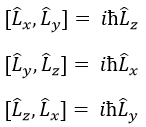Note that Lx and Ly also commute with L2, like Lz does, but their proper values <Lx> and <Ly> equal zero.

The same kind of properties is true for the spin of the electrons with the operators S2 and Sx, Sy, Sz.

To describe the whole system, we have thus a series of equations containing the 5 quantic numbers n, l, ml, s and ms. They form a CSCO (complete set of commuting observables).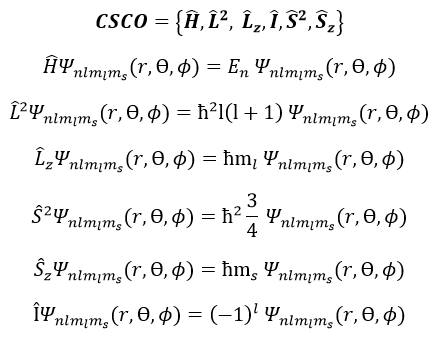Matrix representation

In an orthonormal base of functions {Ψ1, Ψ2,…ΨN}, the operator Â has a matrix representation (Â) which is a matrix composed of operators Âij such as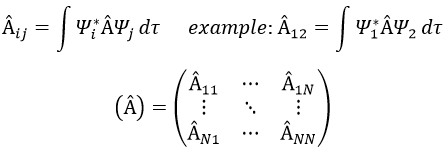If Ψ12, the result would have been the proper value of Ψ1 if Ψ1 is a proper function of Â. It is usual to use the bra-ket notation of Dirac: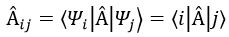The part before the operator is called the bra and the part after the operator is the ket.

If Ψ1 is not a proper function of Â (ÂΨ≠aΨ), then we can find a linear combination (combili) of wave functions such as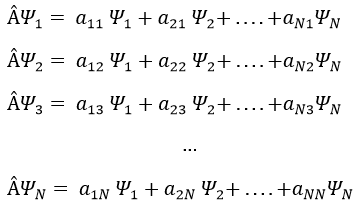or written as matrices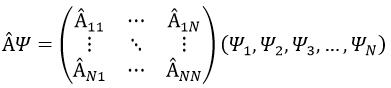Let’s take an example with the operators Lz and L2 and the angular functions Yml that we used before for l=1. The complete base of functions is Y1m={Y11, Y10, Y1-1}. The equations are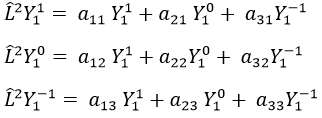The values of aij can easily be found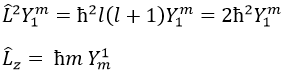The equation for Y11 has no dependence on Y1-1 or Y10. As a result, aij=0 for i≠j and aii=2ћ2.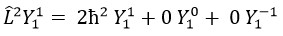The same goes for Y01 and Y11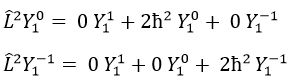Then we have that the matrix representation (L2) of the operator L2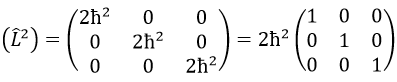This matrix is diagonal, i.e. the components of the matrix that are not on the diagonal are all equal to zero. (Lz) is also diagonal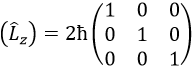The rule is that if the operators are operators of functions that are proper functions of this operator, then the matrix is diagonal. All the operators of the CSCO have a diagonal matrix representation on the base of their common proper functions. Note that it means that Lx and Ly are not part of the CSCO: they don’t commute with Lz and their matrix representation are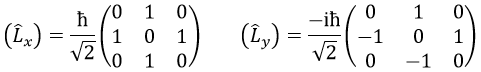It is a direct consequence of the Heisenberg’s uncertainty principle: we cannot know the exact position and speed of a particle at the same time. As a result, L2 cannot commute with all of the Lx, Ly and Lz.

We can define the operators of climbing L+ and of descent L from Lx and Ly.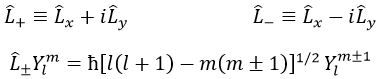For our example with l=1, they are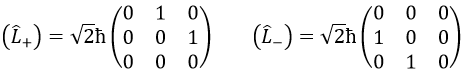Their role is to move from one orbital to another one.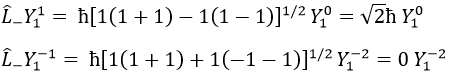As the last line shows it, it is impossible to move out of the system. There is no Y1-2 orbital.